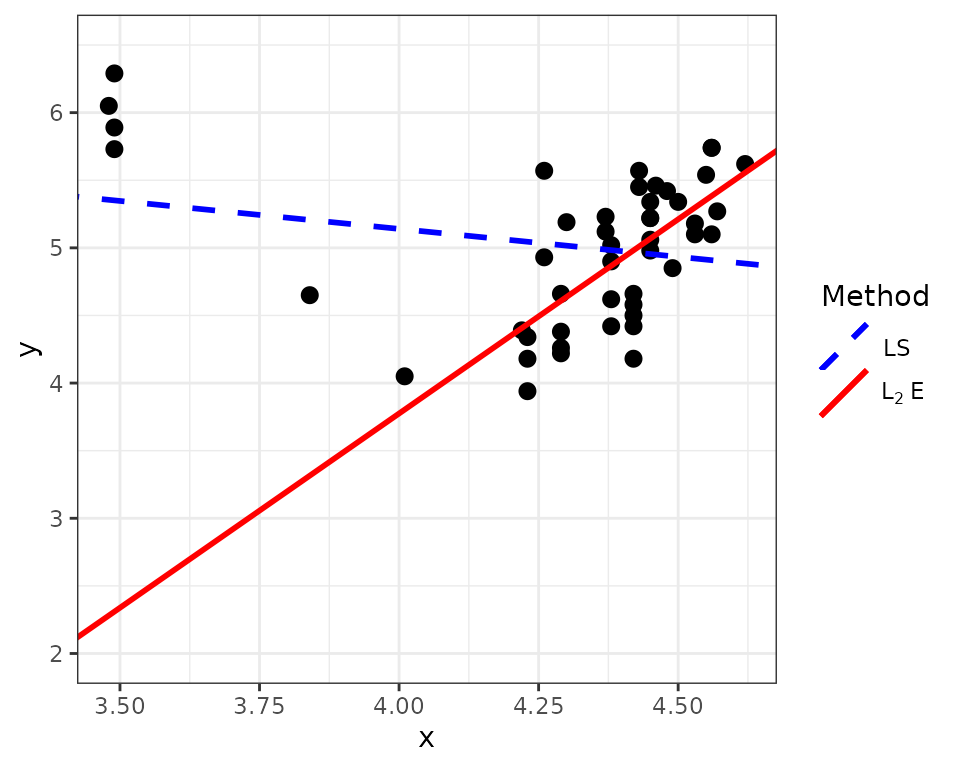$\newcommand{\vbeta}{\boldsymbol{\beta}}$

## Overview

The L2E package implements the computational framework in “A User-Friendly Computational Framework for Robust Structured Regression with the L$$_2$$ Criterion” by Jocelyn T. Chi and Eric C. Chi. This vignette provides code to replicate some examples illustrating the framework in that paper.

## Examples

### Multivariate Regression

The first example provides code to perform multivariate L$$_{2}$$E regression with the Italian bank data from the paper. We begin by loading the bank data from the L2E package.

library(L2E)
y <- bank$y X <- as.matrix(bank[,1:13]) X0 <- as.matrix(cbind(rep(1,length(y)), X)) tau_initial <- 1/mad(y) beta_initial <- matrix(0, 14, 1) The l2e_regression function performs multivariate regression via the L$$_{2}$$ criterion, also called LTE multivariate regression, as described in the paper. It simultaneously obtains an estimate for the coefficient vector $$\vbeta$$ and the precision $$\tau$$. sol <- l2e_regression(y, X0, tau_initial, beta_initial) We can use the estimates for $$\vbeta$$ and $$\tau$$ in sol$beta and sol$tau to identify outlying observations, depicted in blue in the figure below. betaEstimate <- sol$beta
tauEstimate <- sol$tau r <- y - X0 %*% betaEstimate outliers <- which(abs(r) > 3/tauEstimate) l2e_fit <- X0 %*% betaEstimate plot(y, l2e_fit, ylab='Predicted values', pch=16, cex=0.8) points(y[outliers], l2e_fit[outliers], pch=16, col='blue', cex=0.8)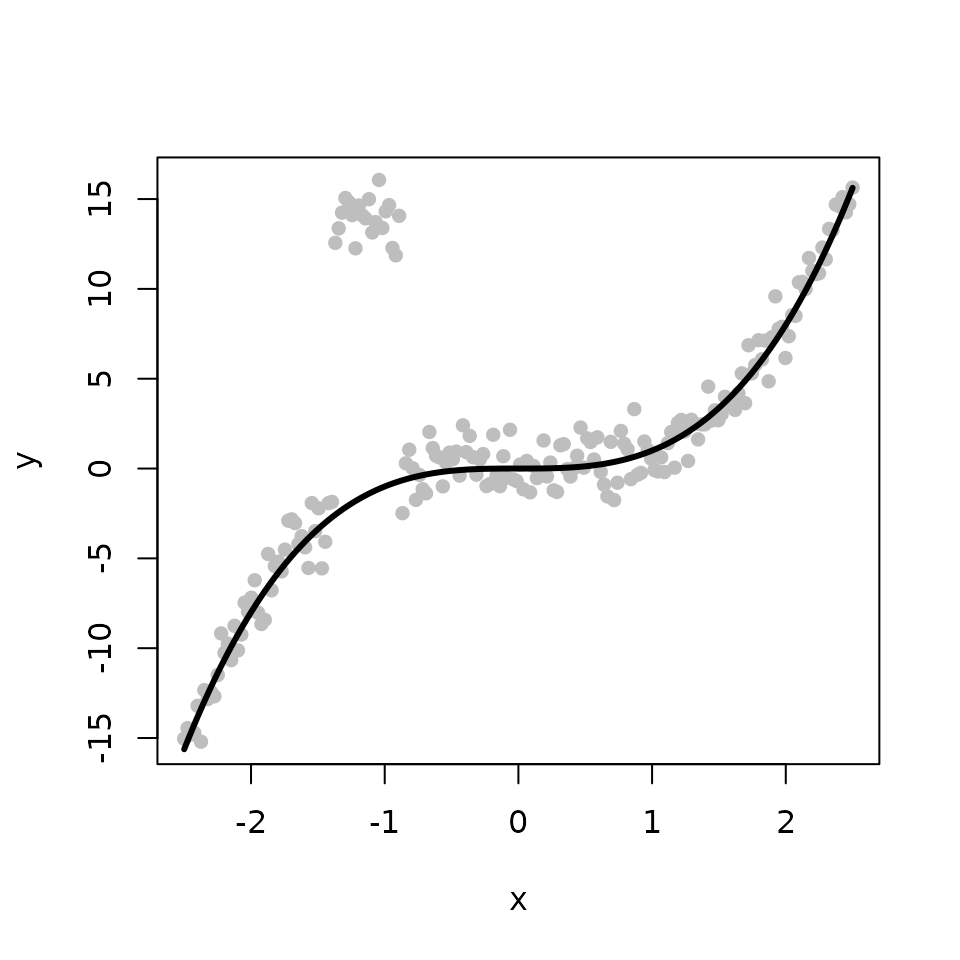For additional figures and discussion on LTE multivariate regression, please refer to the paper. ### Isotonic Regression The second example illustrates how to perform robust isotonic regression via the L$$_{2}$$ criterion, also called LTE isotonic regression. We begin by generating the true fit $$f$$ from a cubic function (depicted by the black line on the figure below). We obtain the observed response by adding some Gaussian noise to $$f$$ (depicted by the gray points on the figure below). set.seed(12345) n <- 200 tau <- 1 x <- seq(-2.5, 2.5, length.out=n) f <- x^3 y <- f + (1/tau)*rnorm(n) plot(x, y, pch=16, col='gray', cex=0.8) lines(x, f, col='black', lwd=3)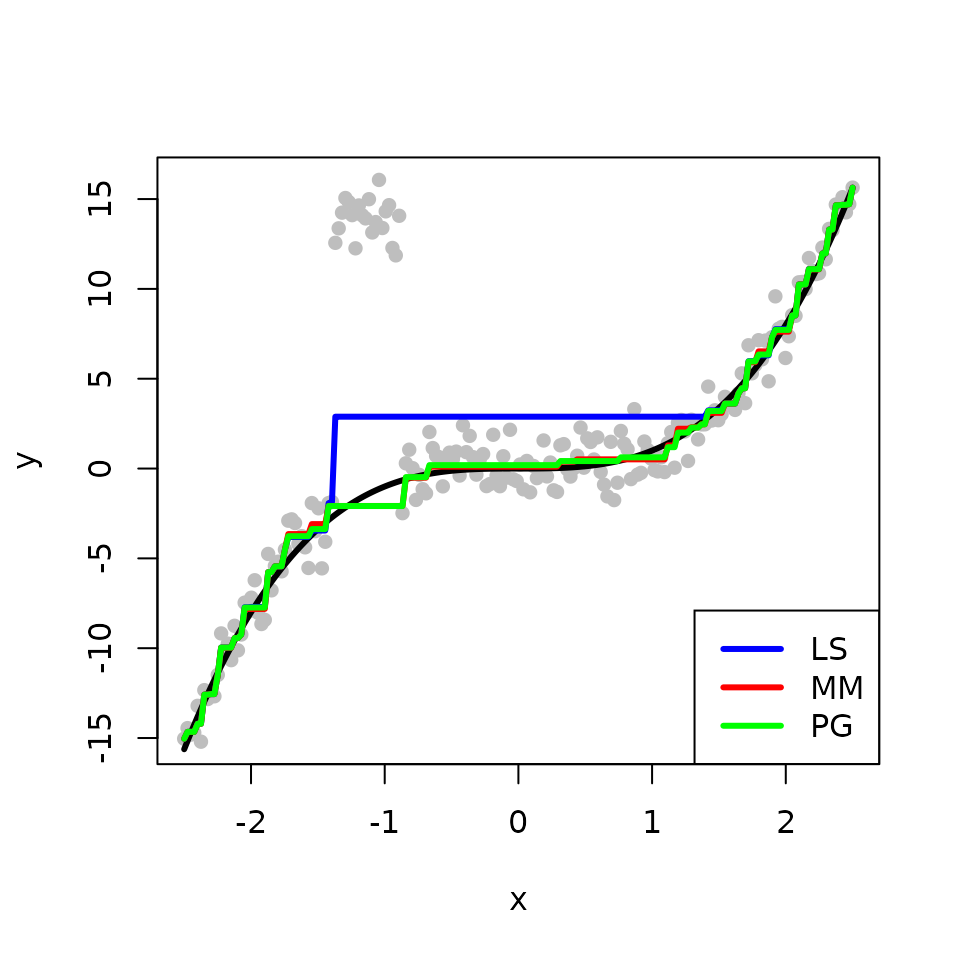The l2e_regression_isotonic function performs robust isotonic regression as described in the paper. We use it to obtain an estimate for the underlying fit $$f$$. The true fit is shown in black while the maximum likelihood estimator (MLE) and the L$$_{2}$$E are shown in red and blue, respectively. In the absence of outliers, the MLE and the L$$_{2}$$E provide similar estimates. tau_initial <- 1 beta_initial <- y sol <- l2e_regression_isotonic(y, beta_initial, tau_initial) isotonic_LTE <- sol$beta

plot(x, y, pch=16, col='gray', cex=0.8)
lines(x, f, col='black', lwd=3)
isotonic_MLE <- gpava(1:n, y)$x lines(x, isotonic_MLE, col='red', lwd=3) lines(x, isotonic_LTE, col='blue', lwd=3)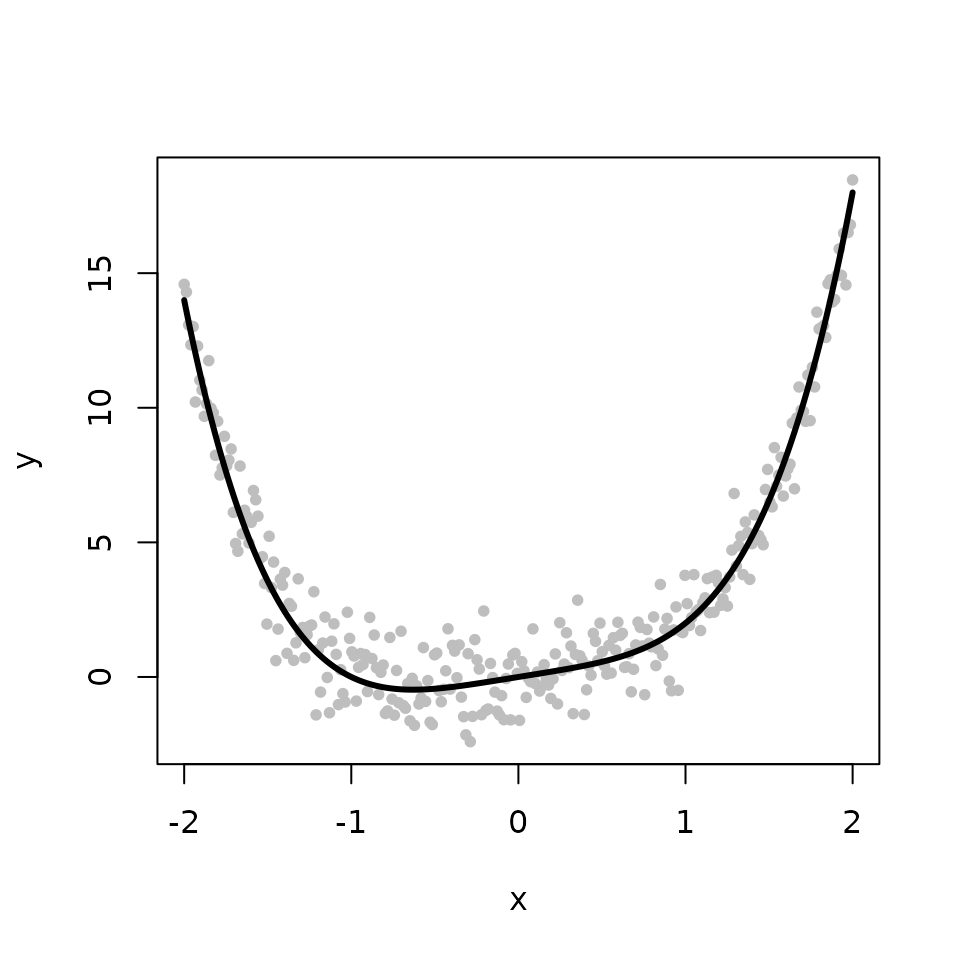Next, we introduce some outliers by perturbing some of the observed responses. ix <- 0:9 y[45 + ix] <- 14 + rnorm(10) plot(x, y, pch=16, col='gray', cex=0.8) lines(x, f, col='black', lwd=3)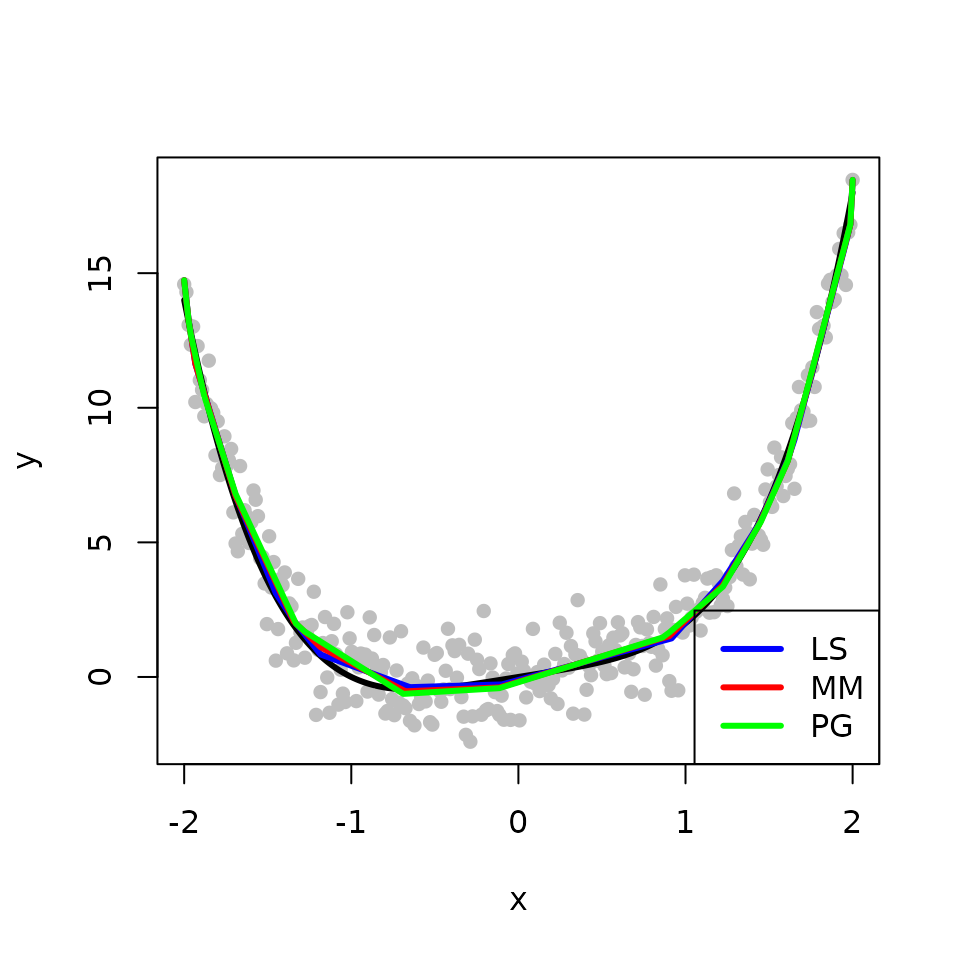We obtain the MLE and the L$$_{2}$$E fits with the outlying points. The L$$_{2}$$E fit is less sensitive to outliers than the MLE in this example. plot(x, y, pch=16, col="gray", cex=0.8) lines(x, f, col='black', lwd=3) isotonic_MLE <- gpava(1:n, y)$x
lines(x, isotonic_MLE, col='red', lwd=3)
sol <- l2e_regression_isotonic(y, beta_initial, tau_initial)
isotonic_LTE <- sol$beta lines(x, isotonic_LTE, col='blue', lwd=3)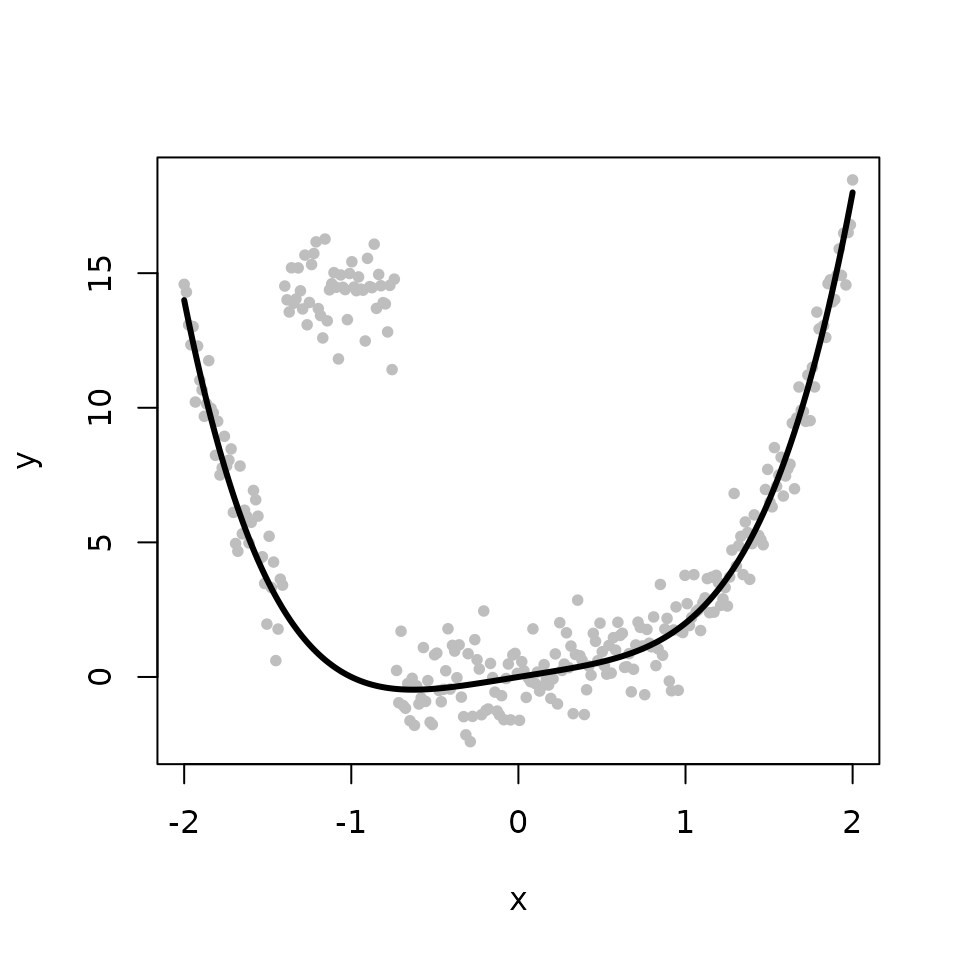### Shape-Restricted Convex Regression The third example illustrates how to perform robust shape-restricted convex regression via the L$$_{2}$$ criterion, also called LTE convex regression. We begin by simulating a convex function $$f$$. The observed response is composed of $$f$$ with some additive Gaussian noise. set.seed(12345) n <- 200 tau <- 1 x <- seq(-2, 2, length.out=n) f <- x^4 + x y <- f + (1/tau) * rnorm(n) plot(x, y, pch=16, col='gray', cex=0.8) lines(x, f, col='black', lwd=3)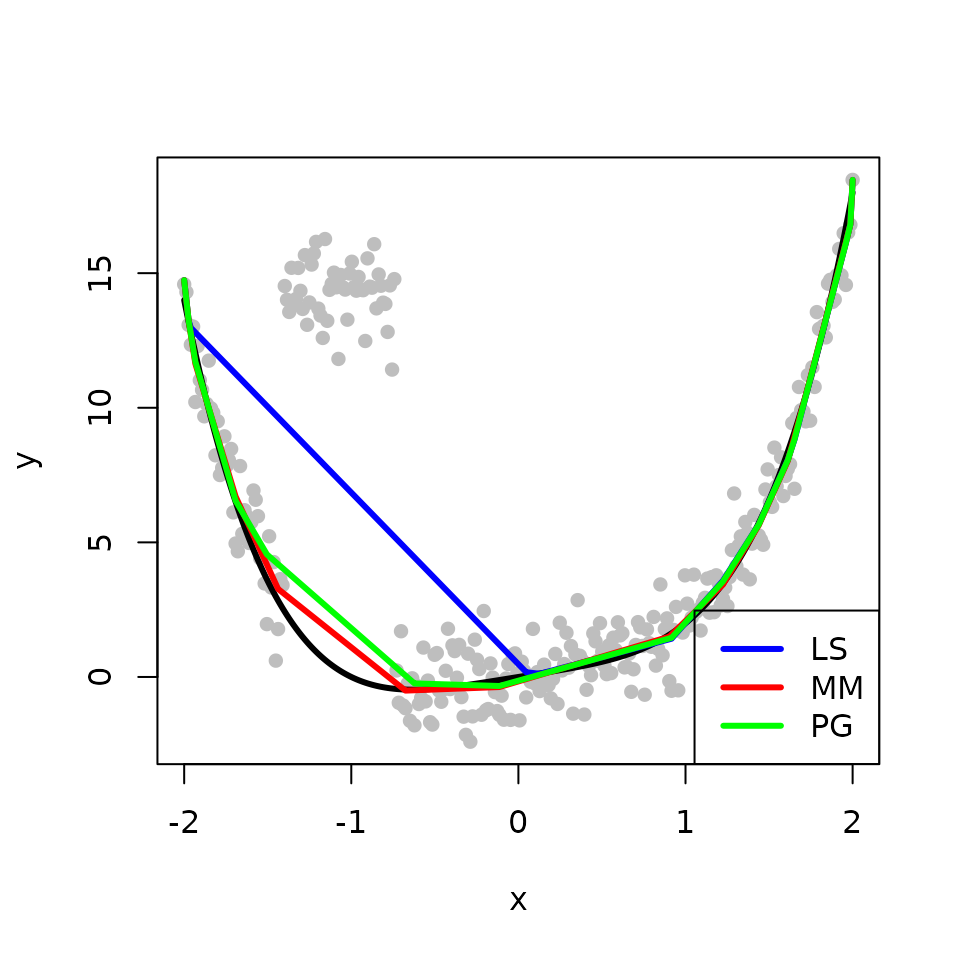The l2e_regression_convex function performs robust convex regression via the L$$_{2}$$ criterion as described in the paper. We use it to obtain an estimate for the underlying convex function $$f$$. The true fit is shown in black while the MLE and the L$$_{2}$$E are shown in red and blue, respectively. In the absence of outliers, the MLE and the L$$_{2}$$E again provide similar estimates. tau_initial <- 1 beta_initial <- y sol <- l2e_regression_convex(y, beta_initial, tau_initial) convex_LTE <- sol$beta

plot(x, y, pch=16, col='gray', cex=0.8)
lines(x, f, col='black', lwd=3)
convex_MLE <- fitted(cobs::conreg(y, convex=TRUE))
lines(x, convex_MLE, col='red', lwd=3)
lines(x, convex_LTE, col='blue', lwd=3)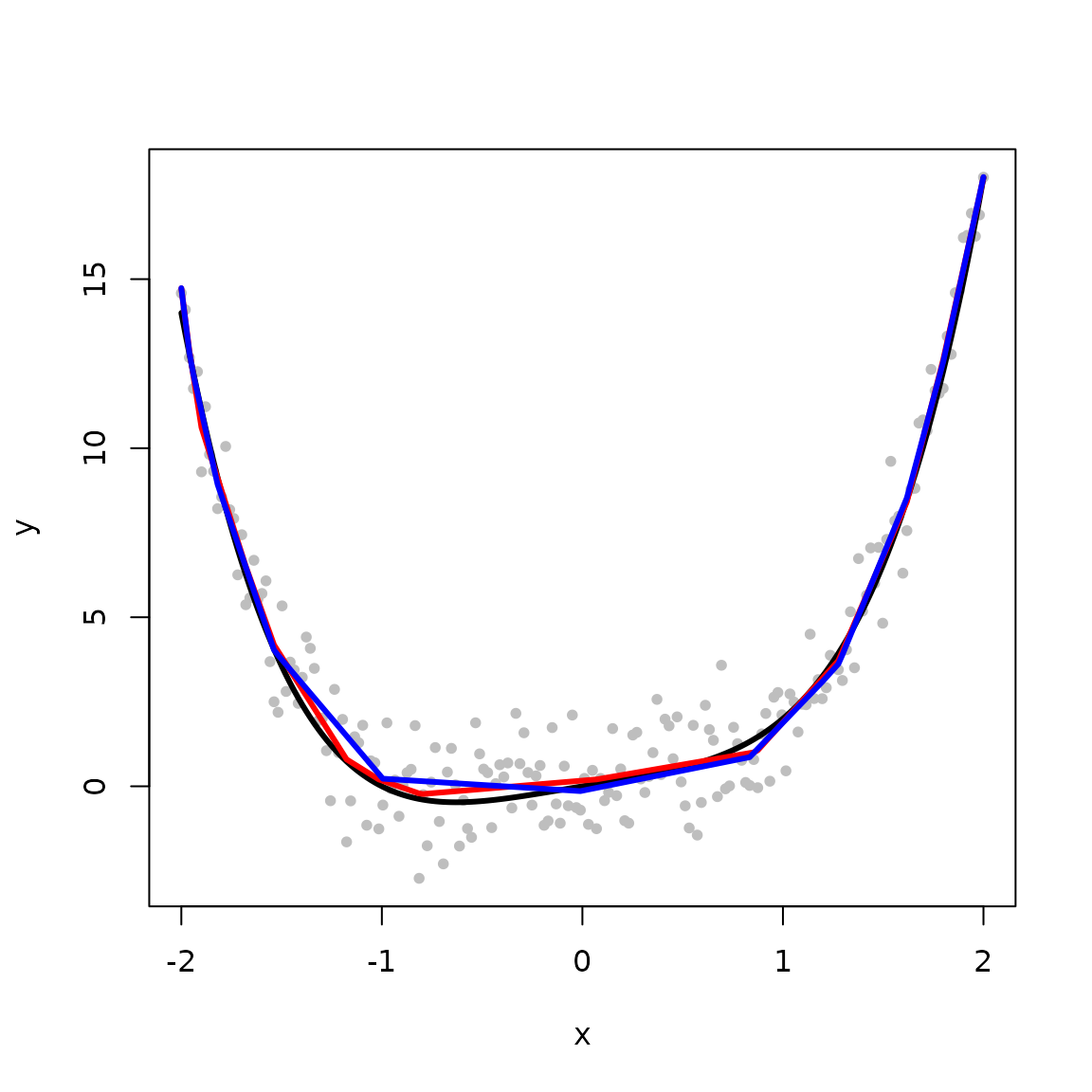Next, we again introduce some outlying points.

ix <- 0:9
y[45 + ix] <- 14 + rnorm(10)

plot(x, y, pch=16, col='gray', cex=0.8)
lines(x, f, col='black', lwd=3)We obtain the MLE and the L$$_{2}$$E fits with the outlying points. The L$$_{2}$$E fit is also less sensitive to the outliers than the MLE in this example.

plot(x, y, pch=16, col='gray', cex=0.8)
lines(x, f, col='black', lwd=3)
convex_MLE <- fitted(cobs::conreg(y, convex=TRUE))
lines(x, convex_MLE, col='red', lwd=3)
sol <- l2e_regression_convex(y, beta_initial, tau_initial)
convex_LTE <- sol\$beta
lines(x, convex_LTE, col='blue', lwd=3)